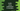# Kotlin development tutorial - Array in Kotlin### Introduction :

The arrays in Kotlin is defined by the Array class. This tutorial will show you how to create an array and how to access array elements in Kotlin with examples.

### How to access an element in Kotlin Array :

Accessing an element is same as other programming languages. You can use myArray[index] to access the element at index position for an array myArray. Index always starts from 0. Examples explaining below are using this method for accessing elements using an index.

Mainly two different methods are available to create one array in Kotlin: using the array constructor and by using one predefined method. Let me show each process one by one :

### Using Array constructor :

The array constructor takes two arguments, first one is the size of the array and the second one is a lambda function that defines the value for a position . For example ,

``````fun main(args: Array) {
val arr = Array(6, { i -> i * i })

for (i in arr.indices) {
println(arr[i])
}
}
``````

It will print :

``````0
1
4
9
16
25
``````

We have passed { i -> i * i } as the second argument to the Array constructor. It says that if the position is i, set value i * i for that position. So, for position 0, the value is 0, for position 3, the value is 9 etc.

### Using predefined arrayOf() function :

Kotlin has one predefined function called arrayOf() to create an array. For example :

``````fun main(args: Array) {
val arr = arrayOf(1,2,3,4,5);

for (i in arr.indices) {
println(arr[i])
}
}
``````

It will create one array of numbers 1,2,3,4 and 5. How about the following one :

``````fun main(args: Array) {
val arr = arrayOf(1,2,3,4,"five",6.25);

for (i in arr.indices) {
println(arr[i])
}
}
``````

It will work. The output array will contain elements of different types. We can also specify the type of element that can be passed to arrayOf() function :

``````fun main(args: Array) {
val arr = arrayOf(1,2,3,4,5);

for (i in arr.indices) {
println(arr[i])
}
}
``````

Here, we have mentioned that the array will contain only Int elements. So, if you will try to add one different type of element here like the second example above, it will throw one compile time error. Kotlin also has specialized class for specific type of array. For example, to create one int array, you can use intArrayOf() method. Other similar methods : byteArrayOf(), shortArrayOf(), longArrayOf() etc.